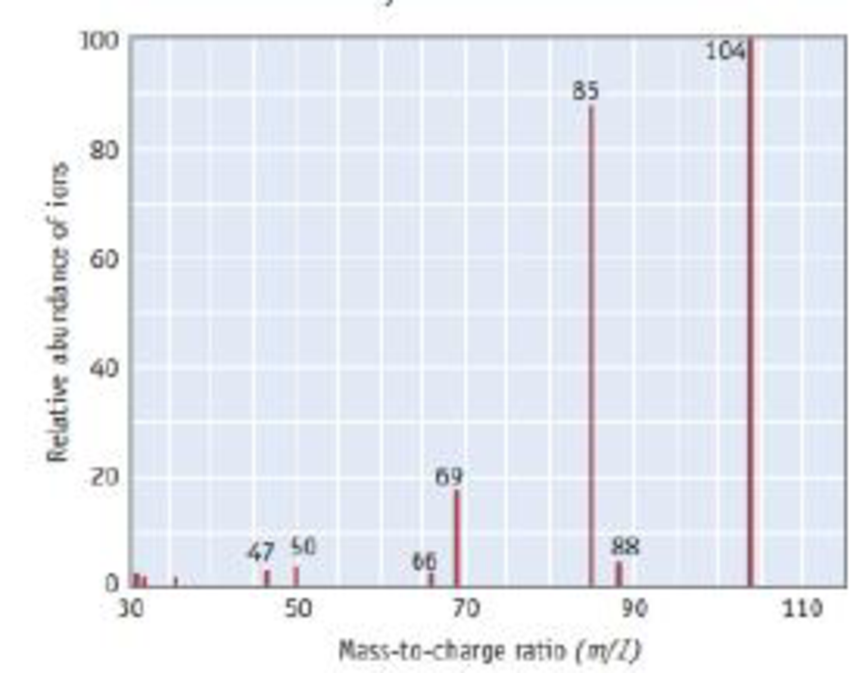Chapter 2, Problem 98PS

Chapter
Section
Textbook Problem

The mass spectrum of phosphoryl chloride. POF3, is illustrated here. (a) Identify the cation fragment at a m/Z ratio of 85. (b) Identify the cation fragment at a m/Z ratio of 69. (c) Which two peaks in the mass spectrum provide evidence that the oxygen atom is connected to the phosphorus atom and is not connected to any of the three fluorine atoms?(a)

Interpretation Introduction

Interpretation:

For the mass spectrum of phosphoryl fluoride, the cations at a m/Z ratio of 85 has to be identified.

Concept introduction:

Mass spectroscopy: It is a form of spectroscopic technique which is used for the elucidation of the molecules, depending upon the mass of the molecule.

Parent ion peak: It is defined as the prominent peak in the mass spectrum of the molecule which represents the not fragmented molecular ion in the given molecule with greater m/z value.

Base peak: It is the tallest peak in the spectrum.

POF3 ionize to form positive ions. Some of them fragment into smaller ions.

Explanation

The prominent peak (POF3+) shows 104 m/Z ratio, so the m/Z ratio of 85 is matching with the m/Z ratio of POF2

(b)

Interpretation Introduction

Interpretation:

For the mass spectrum of phosphoryl fluoride, the cations at a m/Z ratio of 65 has to be identified

Concept introduction:

Mass spectroscopy: It is a form of spectroscopic technique which is used for the elucidation of the molecules, depending upon the mass of the molecule.

Parent ion peak: It is defined as the prominent peak in the mass spectrum of the molecule which represents the not fragmented molecular ion in the given molecule with greater m/z value.

Base peak: It is the tallest peak in the spectrum.

POF3 ionize to form positive ions. Some of them fragment into smaller ions.

(c)

Interpretation Introduction

Interpretation:

For the mass spectrum of phosphoryl fluoride, the bonding of oxygen atoms connected to phosphorus are needed to be explained.

Concept introduction:

Mass spectroscopy: It is a form of spectroscopic technique which is used for the elucidation of the molecules, depending upon the mass of the molecule.

Parent ion peak: It is defined as the prominent peak in the mass spectrum of the molecule which represents the not fragmented molecular ion in the given molecule with greater m/z value.

Base peak: It is the tallest peak in the spectrum.

POF3 ionize to form positive ions. Some of them fragment into smaller ions.

Still sussing out bartleby?

Check out a sample textbook solution.

See a sample solution

The Solution to Your Study Problems

Bartleby provides explanations to thousands of textbook problems written by our experts, many with advanced degrees!

Get Started

Find more solutions based on key concepts